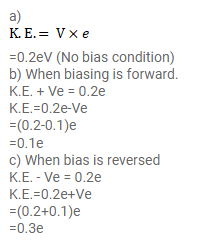# The potential barrier existing across an unbiased p-n junction is

Question:

The potential barrier existing across an unbiased p-n junction is $0.2$ volt. What minimum kinetic energy a hole should have to diffuse from the p-side to the $n$-side if

(a) the junction is unbiased,

(b) the junction is forward biased at $0.1$ volt and

(c) the junction is reverse-biased at $0.1$ volt?

Solution: